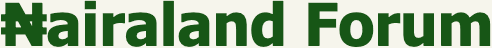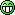#Welcome, Guest: Register On Nairaland / LOGIN! / Trending / Recent / New
Stats: 2,908,528 members, 7,014,348 topics. Date: Saturday, 13 August 2022 at 12:55 AM

## Please, Who Can Assist With The Solution To This Physics / Maths Problem - Education - Nairaland

 Please, Who Can Assist With The Solution To This Physics / Maths Problem by sureteeboy(m): 8:48am On Aug 12, 2020 A small particle moving with a uniform acceleration a, covers distances X and Y in the first two equal and consecutive intervals of time t. Show that a = (Y-X) / t²I tried simplifying this using the 3 equations of motion, but the answer I'm getting is quite different from what was required to proveCc MrShape Femi4 Martinez39s Re: Please, Who Can Assist With The Solution To This Physics / Maths Problem by Martinez39s(m): 9:53am On Aug 12, 2020 Let the two equal time intervals for which the particle covered distances X and Y be [ t1 , t2 ] and [ t1 , t2 ] respectively. It follows thatT = t2 - t1 = t3 - t2 ——— (1) The antiderivative of a(t) = a with respect to t is v(t) = at + C. C is the initial velocity. Since X is the distance traveled in the first interval, X is the definite integral of v(t) with respect to t from t1 to t2. Similarly, Y is the definite integral of v(t) with respect to t from t2 to t3. When you solve the integrals rightly using t1 , t2 , & t3 as the limits of integration, you haveX = ½a(t2)² + Ct2 - ½a(t1)² - Ct1. Y = ½a(t3)² + Ct3 - ½a(t2)² - Ct2. We you solve Y - X and simplify using eq (1), you getY - X = ¼a(t3 - t2)² = ¼a(2T)² NB: In your question, T is written as t. 4 Likes Re: Please, Who Can Assist With The Solution To This Physics / Maths Problem by femi4: 10:16am On Aug 12, 2020 Solved 1 Like Re: Please, Who Can Assist With The Solution To This Physics / Maths Problem by Loris576(m): 10:16am On Aug 12, 2020 Nice one boss 1 Like Re: Please, Who Can Assist With The Solution To This Physics / Maths Problem by Loris576(m): 10:17am On Aug 12, 2020 Martinez39s:Let the two equal time intervals for which the particle covered distances X and Y be [ t1 , t2 ] and [ t1 , t2 ] respectively. It follows thatT = t2 - t1 = t3 - t2 ——— (1) The antiderivative of a(t) = a with respect to t is v(t) = at + C. C is the initial velocity. Since X is the distance traveled in the first interval, X is the definite integral of v(t) with respect to t from t1 to t2. Similarly, Y is the definite integral of v(t) with respect to t from t2 to t3. When you solve the integrals rightly using t1 , t2 , & t3 as the limits of integration, you haveX = ½a(t2)² + Ct2 - ½a(t1)² - Ct1. Y = ½a(t3)² + Ct3 - ½a(t2)² - Ct2. We you solve Y - X and simplify using eq (1), you getY - X = ¼a(t³ - t2)² = ¼a(2T)² NB: In your question, T is written as t.NiceFurther conclude it 1 Like Re: Please, Who Can Assist With The Solution To This Physics / Maths Problem by peppo4live: 10:28am On Aug 12, 2020 Solution:distance (d1) = Xdistance (d2) = Ytime = t1 = t2 = t (equal and consecutive intervals of time)initial velocity = d1/t = X/tfinal velocity = d2/t = Y/tAcceleration (a) = (final velocity - initial velocity)/tPutting X/t and Y/t into the formula for accelerationWe have...Acceleration = (Y/t - X/t)/tFind the LCM inside the bracketWe... (Y-X)/t ÷ t (Y-X)/t × 1/tAcceleration = (Y - X) / t² Proved and shown 4 Likes Re: Please, Who Can Assist With The Solution To This Physics / Maths Problem by Martinez39s(m): 10:38am On Aug 12, 2020 Loris576:NiceFurther conclude it lol. Y - X = ¼a(4T²) = aT²This means a² = (Y - X)/T² 1 Like Re: Please, Who Can Assist With The Solution To This Physics / Maths Problem by Loris576(m): 10:40am On Aug 12, 2020 Martinez39s: lol. Y - X = ¼a(4T²) = aT²This means a² = (Y - X)/T²a** 1 Like 1 Share Re: Please, Who Can Assist With The Solution To This Physics / Maths Problem by sureteeboy(m): 11:08am On Aug 12, 2020 Thanks very much everyoneI was looking at the question from the wrong perspective Re: Please, Who Can Assist With The Solution To This Physics / Maths Problem by femi4: 12:08pm On Aug 12, 2020 peppo4live:Solution:distance (d1) = Xdistance (d2) = Ytime = t1 = t2 = t (equal and consecutive intervals of time)initial velocity = d1/t = X/tfinal velocity = d2/t = Y/tAcceleration (a) = (final velocity - initial velocity)/tPutting X/t and Y/t into the formula for accelerationWe have...Acceleration = (Y/t - X/t)/tFind the LCM inside the bracketWe... (Y-X)/t ÷ t (Y-X)/t × 1/tAcceleration = (Y - X) / t² Proved and shownI like this simple approach 3 Likes Re: Please, Who Can Assist With The Solution To This Physics / Maths Problem by peppo4live: 3:45pm On Aug 12, 2020 femi4:I like this simple approachThanks